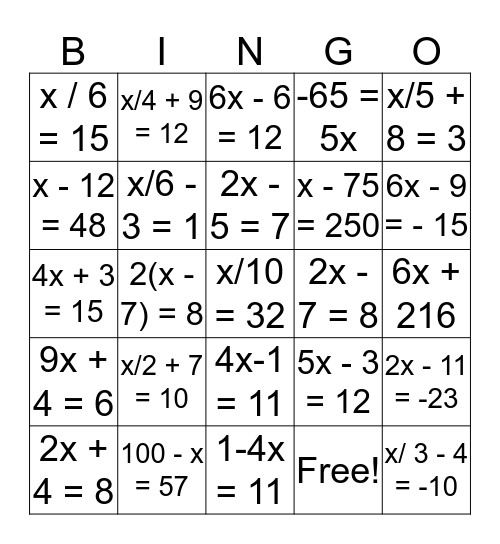# Writing EquationsThis bingo card has 32 words: Free!, 3x + 9 = -6, 2(x - 7) = 8, 9(x + 4 ) = 6, 9x + 4 = 6, x/6 - 3 = 1, x/5 + 8 = 3, 2x - 11 = -23, x/4 + 9 = 12, x/ 3 - 4 = -10, 2x - 5 = 7, 6x - 6 = 12, x + 17 = 85, x + 4 = 68, 2x - 7 = 8, 2x + 7 = 1, 2x + 4 = 8, 4x + 3 = 15, 4x-1 = 11, x/2 + 7 = 10, 5x - 3 = 12, x + 16 = 45, 6x + 216, 100 - x = 57, x/10 = 32, -65 = 5x, x - 75 = 250, x - 12 = 48, x / 6 = 15, 1-4x = 11, x/3 - 5 = -7 and 6x - 9 = - 15.

## Play Online